# 1 Summary

Multi-omics data from the same set of samples contain complementary information and may provide a more accurate and holistic view of the biological system consisting of different interconnected molecular components. Hence, a computational framework to infer regulatory relationships by integrating multiple modalities is one of the most relevant and challenging problems in systems biology.

IntOMICS is an efficient integrative framework based on Bayesian networks. IntOMICS systematically analyses gene expression (GE), DNA methylation (METH), copy number variation (CNV) and biological prior knowledge (B) to infer regulatory networks. IntOMICS complements the missing biological prior knowledge by so-called empirical biological knowledge (empB), estimated from the available
experimental data.

An automatically tuned MCMC algorithm (Yang and Rosenthal, 2017) estimates model parameters and the empirical biological knowledge. Conventional MCMC algorithm with additional Markov blanket resampling (MBR) step (Su and Borsuk, 2016) infers resulting regulatory network structure consisting of three types of nodes: GE nodes refer to gene expression levels, CNV nodes refer to associated copy number variations, and METH nodes refer to associated DNA methylation probe(s). Regulatory networks derived from IntOMICS provide deeper insights into the complex flow of genetic information. IntOMICS is a powerful resource for exploratory systems biology and can provide valuable insights into the complex mechanisms of biological processes that has a vital role in personalised medicine.

IntOMICS takes as input MultiAssayExperiment or named list with:

1. gene expression matrix,
2. associated copy number variation matrix sampled from the same individuals (optional),
3. associated DNA methylation matrix of beta-values sampled from the same individuals (optional), and
4. the biological prior knowledge with information on known interactions among molecular features (optional; highly recommended).

The resulting regulatory network structure contains the edge weights $$w_i$$ representing the empirical frequency of given edge over samples of network structures from two independent MCMC simulations.

For further details about the IntOMICS algorithm, its performance and benchmark analysis, see manuscript Pacinkova & Popovici, 2022.

Projects such as The Cancer Genome Atlas aim to catalogue multi-omics data from a large number of samples. Bioconductor packages such as curatedTCGA enable to download and efficiently collect these multi-omics data. R-packages such as IntOMICS, MOFA2, and cosmosR can provide a complementary understanding of hidden interplay between multi-omics dataset and deciphering the complexity of the biological system.

IntOMICS framework is demonstrated using colon cancer data from the curatedTCGA package. However, it is not limited to any particular omics data or phenotype.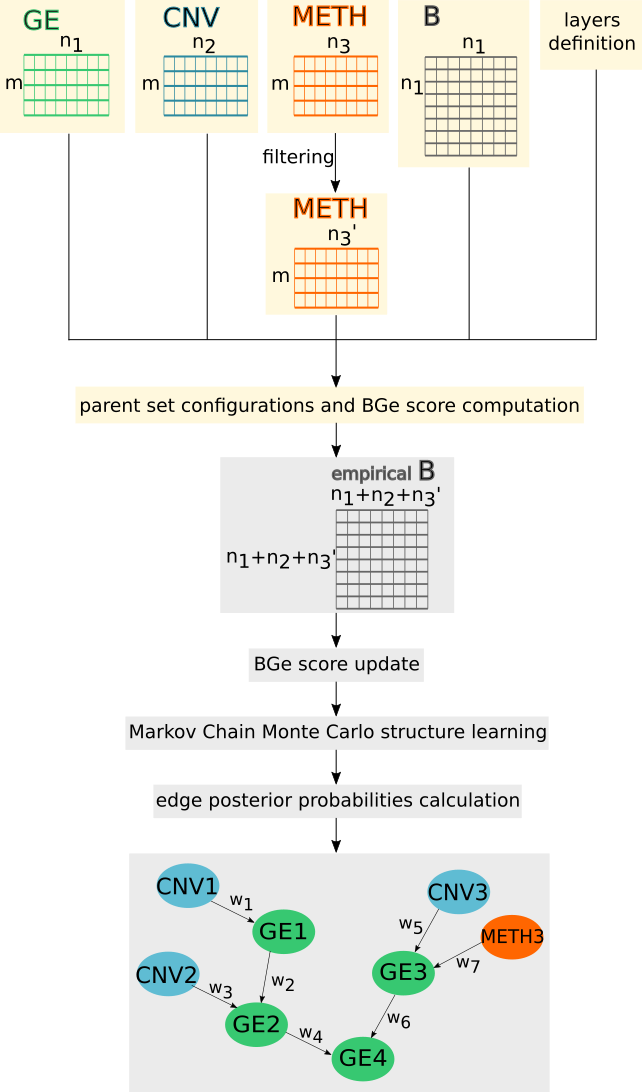IntOMICS framework

# 2 Installation

# bioconductor install
if (!requireNamespace("BiocManager", quietly = TRUE))
install.packages("BiocManager")
BiocManager::install("IntOMICS")

# install the newest (development) version from GitHub
# install.packages("remotes")
remotes::install_github("anna-pacinkova/IntOMICS")  

# 3 Usage

This tutorial will show you how to use the IntOMICS package
with a toy example. The example dataset consisting of processed gene expression, DNA methylation (Illumina Infinium HumanMethylation450 BeadChip) and copy number variation is from the TCGA data portal (https://portal.gdc.cancer.gov/): primary colon cancer samples (COAD) with microsatellite instability (MSI). However,
the approach is not limited to any phenotype. We choose the set of 8 genes from the KEGG Colorectal cancer pathway(https://www.genome.jp/pathway/hsa05210).

## 3.1 Part 1: Input data

library(IntOMICS)

suppressPackageStartupMessages({
library(TCGAutils)
library(bnlearn)
library(bnstruct)
library(matrixStats)
library(parallel)
library(RColorBrewer)
library(bestNormalize)
library(igraph)
library(gplots)
library(methods)
library(ggraph)
library(ggplot2)})
## Warning: replacing previous import 'utils::findMatches' by
## 'S4Vectors::findMatches' when loading 'AnnotationDbi'

IntOMICS framework takes as input:

1. gene expression matrix $$GE$$ ($$m$$ x $$n_1$$) with microarray intensities or RNA-seq count data transformed into a continuous domain ($$m$$ samples and $$n_1$$ features)

2. associated copy number variation matrix $$CNV$$ ($$m$$ x $$n_2$$) with continuous segment mean values derived for each gene ($$n_2 \leq n_1$$),

3. associated DNA methylation matrix of beta-values $$METH$$ ($$m$$ x $$n_3$$),

4. data.frame including all known interactions between molecular features (information from public available databases such as KEGG (Ogata et al., 1999) or REACTOME (Wu & Haw, 2017)). Any other source of prior knowledge can be used. (IntOMICS is designed to run even if prior knowledge is not available. However, we highly recommend to use it.)

5. logical matrix with known transcription factors (TFs) and its targets (information from public available databases such as ENCODE (ENCODE Project Consortium, 2004). Any other source can be used. (IntOMICS is designed to run even if any TF-target interaction is not available.)

All data matrices are sampled from the same individuals.

We use curatedTCGA package to obtain publicly available toy dataset from The Cancer Genome Atlas (TCGA):

coad_SE <- curatedTCGAData("COAD",
c("RNASeq2GeneNorm_illuminahiseq",
"Methylation_methyl450","GISTIC_AllByGene"),
version = "2.0.1", dry.run = FALSE)

## keep NA for Methylation data
coad_ma <- subsetByColData(coad_SE, coad_SE$MSI_status == "MSI-H" | is.na(coad_SE$MSI_status))
# choose random 50 samples for illustration
random_samples <- sample(names(which(table(sampleMap(coad_ma)$primary)==3)),50) coad_ma <- subsetByColData(coad_ma, random_samples) rownames(coad_ma[["COAD_GISTIC_AllByGene-20160128"]]) <- rowData(coad_ma[["COAD_GISTIC_AllByGene-20160128"]])[["Gene.Symbol"]] data(list=c("gene_annot","annot")) rowselect <- list(gene_annot$gene_symbol, gene_annot$gene_symbol, unlist(annot)) names(rowselect) <- names(coad_ma) omics <- coad_ma[rowselect, , ] names(omics) <- c("cnv","ge","meth") Available omics data are saved in a MultiAssayExperiment object including gene expression (GE) of 8 genes + copy number variation (CNV) of 8 genes + beta value of 9 DNA methylation (METH) probes: t(assay(omics[["ge"]]))[1:5,1:5] ## WNT2B FZD2 DVL3 GSK3B AXIN1 ## TCGA-AU-6004-01A-11R-1723-07 18.8040 149.5572 1942.495 1388.871 1151.853 ## TCGA-A6-6649-01A-11R-1774-07 112.5727 63.1428 2296.739 1407.957 1343.538 ## TCGA-F4-6809-01A-11R-1839-07 32.2034 79.6610 2177.966 1397.881 2871.610 ## TCGA-F4-6703-01A-11R-1839-07 5.7186 274.8761 2388.486 1163.553 945.101 ## TCGA-D5-6898-01A-11R-1928-07 28.7695 45.3673 2111.978 1542.120 1558.718 These values correspond to normalised RNA-seq data (in this case transcripts per million (TPM)). However, the user is not limited to this platform. Another assay, such as microarray data, can be used. t(assay(omics[["cnv"]]))[1:5,1:5] ## WNT2B FZD2 DVL3 GSK3B AXIN1 ## TCGA-AU-6004-01A-11D-1717-01 "0.004" "0.005" "-0.000" "-0.002" "-0.019" ## TCGA-A6-6649-01A-11D-1770-01 "-0.028" "-0.167" "0.023" "-0.000" "-0.087" ## TCGA-F4-6809-01A-11D-1834-01 "-0.088" "-0.502" "0.003" "0.000" "0.557" ## TCGA-F4-6703-01A-11D-1834-01 "0.003" "-0.031" "-0.063" "-0.063" "0.034" ## TCGA-D5-6898-01A-11D-1923-01 "0.116" "0.061" "0.096" "0.096" "0.000" These copy number values represent segment mean values equal to $$log_2(\frac{copy-number}{2})$$. In the omics$cnv matrix, define only columns with available CNV data.

t(assay(omics[["meth"]]))[1:5,1:5]
## <5 x 5> matrix of class DelayedMatrix and type "double":
##                              cg14479617 cg12588208 cg27308245 cg26525629
## TCGA-AU-6004-01A-11D-1721-05  0.9241650  0.9210813  0.9269372  0.9347682
## TCGA-A6-6649-01A-11D-1772-05  0.9330947  0.9297589  0.8916435  0.9439792
## TCGA-F4-6809-01A-11D-1837-05  0.9244791  0.9336816  0.8553135  0.9247805
## TCGA-F4-6703-01A-11D-1837-05  0.9019002  0.8821964  0.8578079  0.9233826
## TCGA-D5-6898-01A-11D-1926-05  0.9392662  0.9012298  0.8879660  0.9227228
##                              cg04571584
## TCGA-AU-6004-01A-11D-1721-05  0.4745638
## TCGA-A6-6649-01A-11D-1772-05  0.4717381
## TCGA-F4-6809-01A-11D-1837-05  0.5936627
## TCGA-F4-6703-01A-11D-1837-05  0.6383315
## TCGA-D5-6898-01A-11D-1926-05  0.4288711

These values represent DNA methylation beta values of given probes (defined by IDs).

!!Please, be sure that samples are in the same order across all modalities!!

IntOMICS is designed to infer regulatory networks even if the copy number variation or DNA methylation data (or both) are not available. In such a case, omics must be a MultiAssayExperiment with gene expression.

If methylation data are available, we have to provide an annotation:

str(annot)
## List of 5
##  $GSK3B : chr [1:2] "cg14479617" "cg12588208" ##$ AXIN1 : chr [1:2] "cg27308245" "cg26525629"
##  $WNT2B : chr "cg04571584" ##$ CTNNB1: chr [1:3] "cg04180460" "cg06626556" "cg02247160"
##  $FZD2 : chr "cg09339219" annot is a named list. Each component of the list is a character vector and corresponds to probe IDs associated with a given gene. We also have to provide a gene annotation table: gene_annot ## entrezID gene_symbol ## 14 EID:7482 WNT2B ## 21 EID:2535 FZD2 ## 34 EID:1857 DVL3 ## 35 EID:2932 GSK3B ## 38 EID:8312 AXIN1 ## 40 EID:1499 CTNNB1 ## 36 EID:324 APC ## 43 EID:6934 TCF7L2 gene_annot is Gene ID conversion table with “entrezID” and “gene_symbol” column names. Entrez IDs are used in for regulatory network inference, gene symbols are used for the final regulatory network visualisation. And finally, the prior knowledge from any source chosen by the user: data("PK") PK ## src_entrez dest_entrez edge_type ## 1 EID:7482 EID:2535 present ## 2 EID:2535 EID:1857 present ## 3 EID:1857 EID:2932 present ## 4 EID:324 EID:1499 present ## 5 EID:324 EID:1857 present ## 6 EID:8312 EID:324 present PK is the data.frame with biological prior knowledge about known interactions between features. Column names are “src_entrez” (the parent node), “dest_entrez” (the child node) and “edge_type” (the prior knowledge about the direct interaction between parent and child node; the allowed values are “present” or “missing”). data("TFtarg_mat") TFtarg_mat[1:5,1:5] ## EID:1820 EID:466 EID:1386 EID:467 EID:571 ## EID:1 1 0 0 0 0 ## EID:503538 1 0 0 0 0 ## EID:29974 1 0 0 0 0 ## EID:2 1 0 0 0 0 ## EID:144568 0 0 1 0 0 TFtarg_mat is a logical matrix with known transcription factors (TFs) and its targets. TFs are listed in columns, corresponding targets are listed in rows. data("layers_def") layers_def ## omics layer fan_in_ge ## 1 ge 2 3 ## 2 cnv 1 1 ## 3 meth 1 NA layers_def is a data.frame containing: 1. the modality ID (character vector); must be same as names of omics MultiAssayExperiment object, 2. corresponding layer in IntOMICS MCMC scheme (numeric vector); edges from the lowest layer (must be always GE) to the CNV/METH layers are excluded from the set of candidate edges), and 3. maximal number of parents from given layer to GE nodes (numeric vector). ## 3.2 Part 2: Data preprocessing The first step is to define the biological prior matrix and estimate the upper bound of the partition function needed to define the prior distribution of network structures. We also need to define all possible parent set configurations for each node. For each parent set configuration, we compute the energy (needed to define the prior distribution of network structures) and the BGe score (needed to determine the posterior probability of network structures). These functionalities are available through the omics_module function. We can use linear regression to filter irrelevant DNA methylation probes. In such a case, we set the parameter “lm_METH = TRUE”. We can also specify the threshold for the R^2 to choose DNA methylation probes with significant coefficient using argument “r_squared_thres” (default = 0.3), or p-value using “p_val_thres” (default = 0.05). There are several other arguments: “woPKGE_belief” (default = 0.5) refers to the belief concerning GE-GE interactions without prior knowledge, “nonGE_belief” (default = 0.5) refers to the belief concerning the belief concerning interactions of features except GE (e.g. CNV-GE, METH-GE), “TFBS_belief” refers to the belief concerning the TF and its target interaction (default = 0.75). Note that all interactions with belief equal to “woPKGE_belief” in biological prior knowledge will be updated in empirical biological knowledge. OMICS_mod_res <- omics_module(omics = omics, PK = PK, layers_def = layers_def, TFtargs = TFtarg_mat, annot = annot, gene_annot = gene_annot, lm_METH = TRUE, r_squared_thres = 0.3, p_val_thres = 0.1) This function returns several outputs: names(OMICS_mod_res) ##  "pf_UB_BGe_pre" "B_prior_mat" "annot" ##  "omics" "layers_def" "omics_meth_original" 1. OMICS_mod_res$pf_UB_BGe_pre is a list that contains:
• OMICS_mod_res$pf_UB_BGe_pre$partition_func_UB the upper bound of the partition function for hyperparameter $$\beta = 0$$,
• OMICS_mod_res$pf_UB_BGe_pre$parents_set_combinations all possible parent set configuration for given node,
• OMICS_mod_res$pf_UB_BGe_pre$energy_all_configs_node energy for given parent set configurations,
• OMICS_mod_res$pf_UB_BGe_pre$BGe_score_all_configs_node BGe score for given parent set configurations.
1. OMICS_mod_res$B_prior_mat is a biological prior matrix. 2. OMICS_mod_res$annot contains DNA methylation probes that passed the filter.
3. OMICS_mod_res$omics is a list with gene expression, copy number variation and normalised methylation data (possibly filtered if we use “lm_METH = TRUE”). 4. OMICS_mod_res$omics_meth_original the original methylation data.

## 3.3 Part 3: MCMC simulation

We use the automatically tuned MCMC algorithm (Yang and Rosenthal, 2017) with default setting to estimate model hyperparameter $$\beta$$ and empirical biological knowledge matrix through multiple phases.

1. The first adaptive phase is used to roughly tune the hyperparameter $$\beta$$, more precisely the variance of its proposal distribution.

2. The transient phase is applied to diagnose whether the chain has reached the mode of the target distribution.

3. The second adaptive phase is used to fine-tune the hyperparameter $$\beta$$, the variance of its proposal distribution and to compute the empirical biological prior matrix. Assuming there is no prior knowledge aboit interaction from node i to node j, the prior knowledge about interaction from node i to node j is updated during the second adaptive phase after every conventional edge proposal move (addition, deletion, reversal).
The empirical biological knowledge corresponds to the ratio of acceptance (number of iterations with accepted candidate edge from~node i to~node j) and frequency (number of iterations with proposed candidate edge from~node i to~node j). Reversing an edge is equivalent to deleting the edge and adding the edge in the opposite direction.

4. The empirical biological matrix and the hyperparameter $$\beta$$ determined bythesecond adaptive phase are used in the last sampling phase. In this phase, IntOMICS applies a greedy horizon approach. Three independent paths are executed with a fixed BGe score (except the MBR step). The most probable path is chosen after every 500 iterations.
The conventional MCMC algorithm with additional Markov blanket resampling step (Su and Borsuk, 2016) to infer regulatory network structure consisting of three types of nodes: GE, CNV and METH nodes. Two independent samples of network structures are produced to evaluate the convergence of the Markov chain. Each sample consists of 2*burn_in DAGs. The~resulting samples of DAGs are thinned - discarded all but every thin DAG. The burn-in period and thinning frequency are arbitrary choices.

if(interactive())
{
BN_mod_res_sparse <- bn_module(burn_in = 500,
thin = 50,
OMICS_mod_res = OMICS_mod_res,
minseglen = 5)
}

Because of the MCMC simulation, bn_module function is time-consuming. For the illustration, we have used only short burn-in period of the resulting Markov chain. We recommend to use much longer burn-in period and check trace plots of the MCMC simulation. We recommend to use burn_in = 100.000, thin = 500, and minseglen = 50.000 (for further details see (Pacinkova & Popovici, 2022)). To investigate relevant IntOMICS outputs, use the pre-computed result saved in BN_mod_res R object).
There are two optional arguments: “len” specifies the initial width of the sampling interval for hyperparameter $$\beta$$. However, this parameter will be tuned during the adaptive phases of the MCMC algorithm. “prob_mbr” specifies the probability of the MBR step (default = TRUE). We strongly recommend to use the default setting (for further details on how this argument affects MCMC scheme results, see (Su and Borsuk, 2016)).

Let’s check the outputs of bn_module function:

data("BN_mod_res")
getSlots(class(BN_mod_res))
##       estimated_beta        estimated_len B_prior_mat_weighted
##            "numeric"            "numeric"             "matrix"
##          beta_tuning          CPDAGs_sim1          CPDAGs_sim2
##             "matrix"               "list"               "list"
##                  rms
##            "numeric"
1. estimated_beta(BN_mod_res) Numeric, estimated value of hyperparameter $$\beta$$

2. estimated_len(BN_mod_res) Numeric, estimated width of the sampling interval for hyperparameter $$\beta$$

3. B_prior_mat_weighted(BN_mod_res) Empirical biological knowledge matrix, interactions from the biological prior knowledge and TFs-target interactions are constant (unless “TFBS_belief” is not equal to “woPKGE_belief”).

4. CPDAGs_sim1(BN_mod_res) List of CPDAGs from the first independent MCMC simulation (thinned DAGs from the MCMC simulation converted into CPDAGs, duplicated CPDAGs discarded)

5. CPDAGs_sim2(BN_mod_res) List of CPDAGs from the second independent MCMC simulation (thinned DAGs from the MCMC simulation converted into CPDAGs, duplicated CPDAGs discarded)

6. beta_tuning(BN_mod_res) Matrix of results from adaptive phases that contains hyperparameter $$\beta$$ tuning (trace of hyperparameter $$\beta$$, trace of width of the sampling interval for hyperparameter $$\beta$$

7. rms(BN_mod_res) Numeric, trace of root mean square used for c_rms measure to evaluate the convergence of MCMC simulation

## 3.4 Part 4: MCMC diagnostics

Trace plots provide an important tool for assessing mixing of a Markov chain and
should be inspected carefully. We can generate them using the trace_plots functions:

trace_plots(mcmc_res = BN_mod_res,
burn_in = 10000,
thin = 500,
edge_freq_thres = 0.5)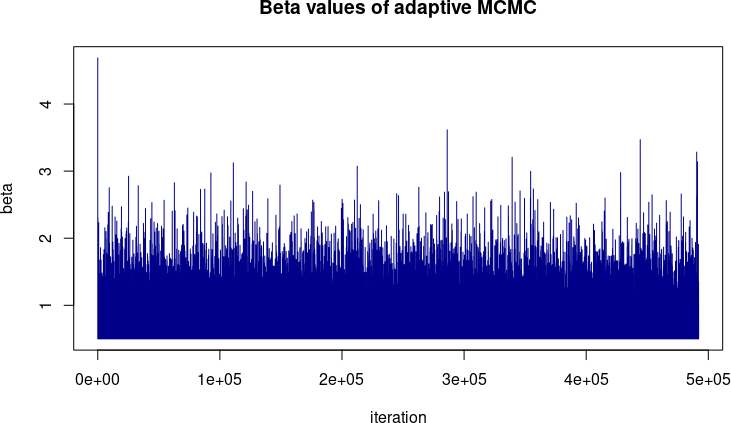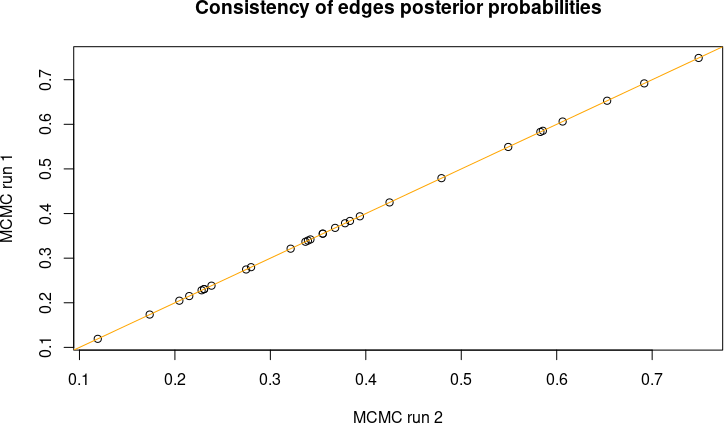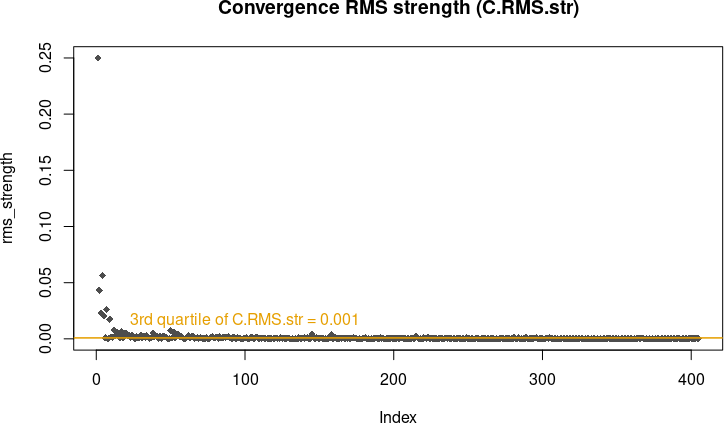The trace_plots function generates the following:

1. trace plot of beta values (we want to explore the sample space many times and avoid flat bits - the chain stays in the same state for too long; in this case, beta value fluctuates around single value, so the Markov chain is mixing well).

2. consistency of edges posterior probabilities in two independent MCMC simulations (scatter plot of the edge weights confidence using two independent MCMC runs; the convergence is determined by the spread of the points around the y=x line; in this case, the edge weights seems to be consistent in two independent simulations).

3. the crms strength for the convergence evaluation (summarizes the spread of the points around the line y=x, for details see (Agostinho et al., 2015) and (Pacinkova & Popovici, 2022)).

The parameter “edge_freq_thres” determines the quantile of all edge weights used to filter only reliable edges (default = NULL, all edges will be considered as present). For illustration, we use quite low edge weights filter to capture interactions between features from different layers. We recommend to use some edge weights filtering, such as 0.75 quantile of all edge weights in the resulting networks using “edge_freq_thres = 0.75”.

## 3.5 Part 5: IntOMICS resulting network structure

Now we use edge_weights and weighted_net functions to define the resulting regulatory network inferred by IntOMICS with specific thresholds:

res_weighted <- edge_weights(mcmc_res = BN_mod_res,
burn_in = 10000,
thin = 500,
edge_freq_thres = 0.5)

weighted_net_res <- weighted_net(cpdag_weights = res_weighted,
gene_annot = gene_annot,
PK = PK,
OMICS_mod_res = OMICS_mod_res,
gene_ID = "gene_symbol",
TFtargs = TFtarg_mat,
B_prior_mat_weighted = B_prior_mat_weighted(BN_mod_res))

The parameter “gene_ID” determines the IDs used in the final network. There are two options: “gene_symbol” (default) or “entrezID”.

We can plot the resulting regulatory network inferred by IntOMICS using ggraph_weighted_net function (node size and label size can be modified):

ggraph_weighted_net(net = weighted_net_res,
node_size = 10,
node_label_size = 4,
edge_label_size = 4)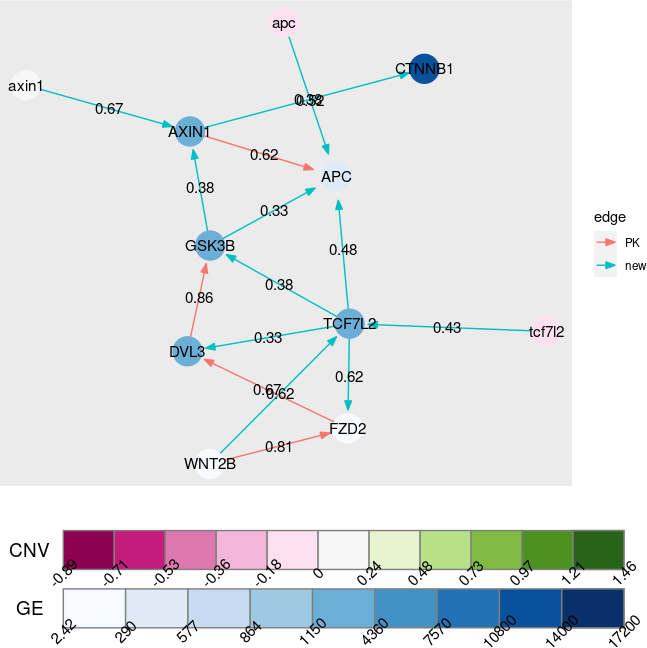Edges highlighted in blue are known from the biological prior knowledge. The edge labels reflect its empirical frequency over the final set of CPDAGs. GE node names are in upper case, CNV node names are in lower case, and METH node names are the same as DNA methylation probe names in omics\$meth matrix. Node colour scales are given by GE/CNV/METH values of all features from the corresponding input data matrix.

We can also change the edge labels to inspect the empirical prior knowledge inferred by IntOMICS using the argument “edge_weights = empB” (default = “MCMC_freq”):

weighted_net_res <- weighted_net(cpdag_weights = res_weighted,
gene_annot = gene_annot,
PK = PK,
OMICS_mod_res = OMICS_mod_res,
gene_ID = "gene_symbol",
edge_weights = "empB",
TFtargs = TFtarg_mat,
B_prior_mat_weighted = B_prior_mat_weighted(BN_mod_res))
ggraph_weighted_net(net = weighted_net_res)